# Converting Pandas Crosstab into Stacked DataFrame

• Last Updated : 11 Jan, 2022

In this article, we will discuss how to convert a pandas crosstab to a stacked dataframe.

A stacked DataFrame is a multi-level index with one or more new inner levels as compared to the original DataFrame. If the columns have a single level, then the result is a series object.

The panda’s crosstab function is a frequency table that shows the relationship between two or more variables by building a cross-tabulation table that computes the frequency among certain groups of data.

Syntax:

pandas.crosstab(index, columns, rownames=None, colnames=None)

Parameters:

• index – array or series or list of array-like objects. this value is used to group in rows
• columns – array or series or list of array-like objects. this value is used to group in columns
• rownames – the name specified here must match the number of row arrays passed.
• colnames – the name specified here must match the number of column arrays passed.

Example:

In this example, we are creating 3 sample arrays namely car_brand, version, fuel_type as shown. Now, we are passing these arrays as the index, columns, and row and column names to the crosstab function as shown.

Finally, crosstab dataframe can also be visualized using the python plot.bar() function

## Python3

 `# import the numpy and pandas package``import` `numpy as np``import` `pandas as pd` `# create three separate arrays namely car_brand,``# version, fuel_type as shown``car_brand ``=` `np.array([``"bmw"``, ``"bmw"``, ``"bmw"``, ``"bmw"``, ``"benz"``, ``"benz"``,``                      ``"bmw"``, ``"bmw"``, ``"benz"``, ``"benz"``, ``"benz"``, ``"benz"``,``                      ``"bmw"``, ``"bmw"``, ``"bmw"``, ``"benz"``, ``"benz"``, ],``                     ``dtype``=``object``)` `version ``=` `np.array([``"one"``, ``"one"``, ``"one"``, ``"two"``, ``"one"``, ``"one"``, ``"one"``,``                    ``"two"``, ``"one"``, ``"one"``, ``"one"``, ``"two"``, ``"two"``, ``"two"``,``                    ``"one"``, ``"two"``, ``"one"``], dtype``=``object``)` `fuel_type ``=` `np.array([``"petrol"``, ``"petrol"``, ``"petrol"``, ``"diesel"``, ``"diesel"``,``                      ``"petrol"``, ``"diesel"``, ``"diesel"``, ``"diesel"``, ``"petrol"``,``                      ``"petrol"``, ``"diesel"``, ``"petrol"``, ``"petrol"``, ``"petrol"``,``                      ``"diesel"``, ``"diesel"``, ],``                     ``dtype``=``object``)` `# use pandas crosstab and pass the three arrays``# as index and columns to create a crosstab table.``cross_tab_data ``=` `pd.crosstab(index``=``car_brand,``                             ``columns``=``[version, fuel_type],``                             ``rownames``=``[``'car_brand'``],``                             ``colnames``=``[``'version'``, ``'fuel_type'``])` `print``(cross_tab_data)` `barplot ``=` `cross_tab_data.plot.bar()`

Output: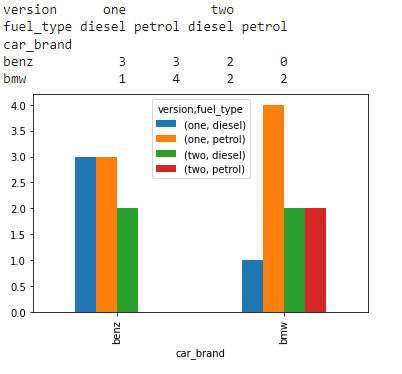## Conversion of  crosstab to stacked dataframe:

Here we are going to specify the number of the levels to be stacked. This will convert based on the axis levels on the particular columns of the pandas DataFrame.

Syntax:

`pandas.DataFrame.stack(level, dropna)`

Parameters:

• level – specifies the levels to be stacked from the column axis to the index axis in the resulting dataframe
• dropna – a bool type. Whether to drop or not the rows in the resulting DataFrame/Series with missing values

Example 1:

Here, We will convert the crosstab to a stacked dataframe. The fuel_type level will be stacked as a column in the resulting dataframe.

## Python3

 `# import the numpy and pandas package``import` `numpy as np``import` `pandas as pd` `# create three separate arrays namely car_brand,``# version, fuel_type as shown``car_brand ``=` `np.array([``"bmw"``, ``"bmw"``, ``"bmw"``, ``"bmw"``, ``"benz"``, ``"benz"``,``                      ``"bmw"``, ``"bmw"``, ``"benz"``, ``"benz"``, ``"benz"``, ``"benz"``,``                      ``"bmw"``, ``"bmw"``, ``"bmw"``, ``"benz"``, ``"benz"``, ],``                     ``dtype``=``object``)` `version ``=` `np.array([``"one"``, ``"one"``, ``"one"``, ``"two"``, ``"one"``, ``"one"``, ``"one"``,``                    ``"two"``, ``"one"``, ``"one"``, ``"one"``, ``"two"``, ``"two"``, ``"two"``,``                    ``"one"``, ``"two"``, ``"one"``], dtype``=``object``)` `fuel_type ``=` `np.array([``"petrol"``, ``"petrol"``, ``"petrol"``, ``"diesel"``, ``"diesel"``,``                      ``"petrol"``, ``"diesel"``, ``"diesel"``, ``"diesel"``, ``"petrol"``,``                      ``"petrol"``, ``"diesel"``, ``"petrol"``, ``"petrol"``, ``"petrol"``,``                      ``"diesel"``, ``"diesel"``, ],``                     ``dtype``=``object``)` `# use pandas crosstab and pass the three``# arrays as index and columns``# to create a crosstab table.``cross_tab_data ``=` `pd.crosstab(index``=``car_brand,``                             ``columns``=``[version, fuel_type],``                             ``rownames``=``[``'car_brand'``],``                             ``colnames``=``[``'version'``, ``'fuel_type'``])` `barplot ``=` `cross_tab_data.plot.bar()` `# use the created sample crosstab data``# to convert it to a stacked dataframe``stacked_data ``=` `cross_tab_data.stack(level``=``1``)` `print``(stacked_data)`

Output: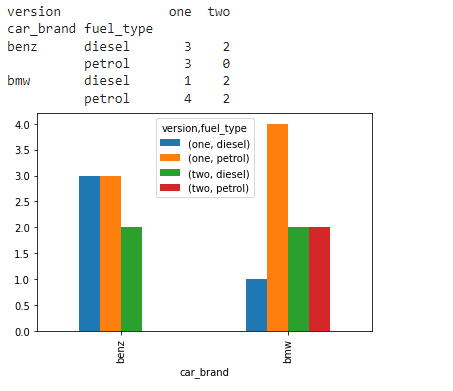Example 2

In this example, we have shown the results for two levels 1 and 2.

## Python3

 `# import the numpy and pandas package``import` `numpy as np``import` `pandas as pd` `# create three separate arrays namely car_brand,``# version, fuel_type as shown``car_brand ``=` `np.array([``"bmw"``, ``"bmw"``, ``"bmw"``, ``"bmw"``, ``"benz"``,``                      ``"benz"``, ``"bmw"``, ``"bmw"``, ``"benz"``, ``"benz"``,``                      ``"benz"``, ``"benz"``, ``"bmw"``, ``"bmw"``, ``"bmw"``,``                      ``"benz"``, ``"benz"``, ], dtype``=``object``)` `version ``=` `np.array([``"one"``, ``"one"``, ``"one"``, ``"two"``, ``"one"``, ``"one"``,``                    ``"one"``, ``"two"``, ``"one"``, ``"one"``, ``"one"``, ``"two"``,``                    ``"two"``, ``"two"``, ``"one"``, ``"two"``, ``"one"``],``                   ``dtype``=``object``)` `fuel_type ``=` `np.array([``"petrol"``, ``"petrol"``, ``"petrol"``, ``"diesel"``,``                      ``"diesel"``, ``"petrol"``, ``"diesel"``, ``"diesel"``,``                      ``"diesel"``, ``"petrol"``, ``"petrol"``, ``"diesel"``,``                      ``"petrol"``, ``"petrol"``, ``"petrol"``, ``"diesel"``,``                      ``"diesel"``, ], dtype``=``object``)` `year_release ``=` `np.array([``2000``, ``2005``, ``2000``, ``2007``, ``2000``, ``2005``,``                         ``2007``, ``2005``, ``2005``, ``2000``, ``2007``, ``2000``,``                         ``2007``, ``2005``, ``2005``, ``2007``, ``2000``],``                        ``dtype``=``object``)` `# use pandas crosstab and pass the three arrays``# as index and columns to create a crosstab table.``cross_tab_data ``=` `pd.crosstab(index``=``car_brand,``                             ``columns``=``[version, fuel_type, year_release],``                             ``rownames``=``[``'car_brand'``],``                             ``colnames``=``[``'version'``, ``'fuel_type'``, ``'year_release'``])` `barplot ``=` `cross_tab_data.plot.bar()` `# use the created sample crosstab data to``# convert it to a stacked dataframe with``# level 1``stacked_data ``=` `cross_tab_data.stack(level``=``1``)`  `barplot ``=` `stacked_data.plot.bar()` `# use the created sample crosstab data to``# convert it to a stacked dataframe with``# level 2``stacked_data ``=` `cross_tab_data.stack(level``=``2``)` `barplot ``=` `stacked_data.plot.bar()`

Output: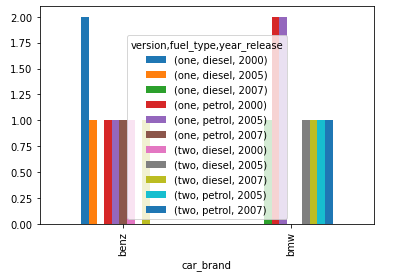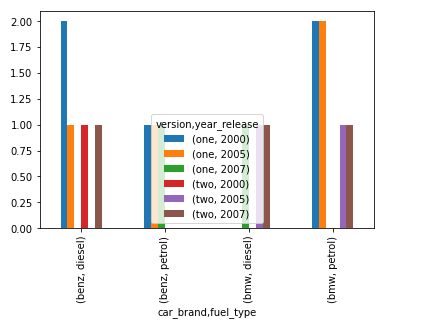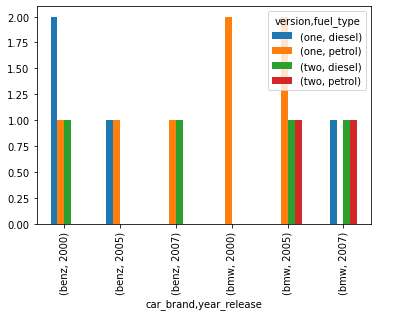My Personal Notes arrow_drop_up